# 配方法

"配方"是……

 ……拿一个像这样的二次方程：把它转化为： ax2 + bx + c = 0 a(x+d)2 + e = 0

 如果你没有时间看下去，我可以告诉你： d = b2a 并且： e = c − b24a

## 配方法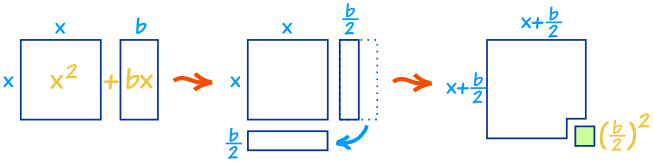x2 + bx可以几乎重排成一个正方形……

……我们可以搭配上(b/2)2完成这个正方形

 x2 + bx + (b/2)2 = (x+b/2)2 "配方"

## 保持平衡

 开始：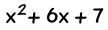（在这里"b" 是 6） 配方：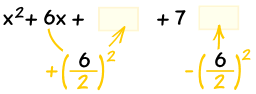同时，减同一项 简化，就做好了。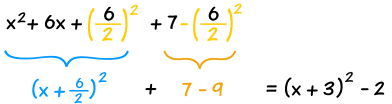x2 + 6x + 7   =   (x+3)2 2

## 捷径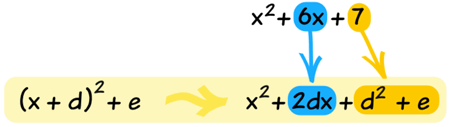• 我们知道6x会成为2dx，所以d一定是 3
• 我们也知道7会成为 d2 + e = 9 + e，所以e 一定是 −2

## 用配方法来解一般二次方程

ax2 + bx + c = 0

x2 + (b/a)x + c/a = 0

## 步骤

• 一、 把所有项除以 ax2的系数）。
• 二、 把常数项 (c/a) 移到方程的右边。
• 三、 左边配方，右边也加同项以保持平衡。

• 四、 取方程两边的平方根。
• 五、 减去左边剩下的数来求x

## 例子

### 例子 1： 解 x2 + 4x + 1 = 0

x2 + 4x = -1

(b/2)2 = (4/2)2 = 22 = 4

x2 + 4x + 4 = -1 + 4
(x + 2)2 = 3

x + 2 = ±√3 = ±1.73（保留2位小数）

x = ±1.73 – 2 = -3.73 or -0.27
 还有一个有趣并且有用的小知识。 做完第三步后，方程是： (x + 2)2 = 3 这方程告诉我们 x2 + 4x + 1 的顶点（转折点）在(-2, -3)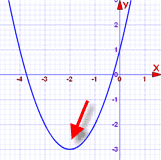### 例子 2：解 5x2 – 4x – 2 = 0

x2 – 0.8x – 0.4 = 0

x2 – 0.8x = 0.4

(b/2)2 = (0.8/2)2 = 0.42 = 0.16

x2 – 0.8x + 0.16 = 0.4 + 0.16
(x – 0.4)2 = 0.56

x – 0.4 = ±√0.56 = ±0.748 （保留3位小数）

x = ±0.748 + 0.4 = -0.348 or 1.148

## 为什么要"配方"？

### 附注： "d"和 "e" 的值

 开始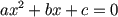除以 a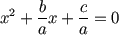把 c/a 移到另一边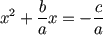每边加 (b/2a)2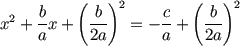"配方"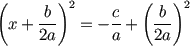把所有项移到…… ……左边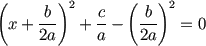……乘以 a，还原 x2的指数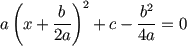我们得到了： a(x+d)2 + e = 0 其中： d = b 2a 并且： e = c − b2 4a 和页顶一样！